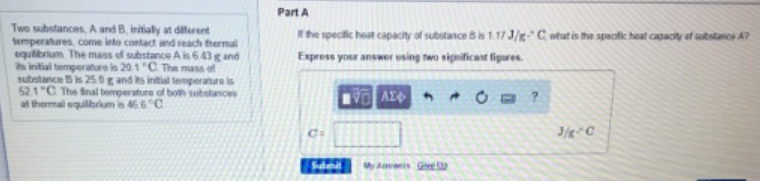# Problem: Two substances, A and B, initially at different temperature, come into contact and reach thermal equilibrium. The mass of substance A is 6.43 g and the initial temperature is 20.1°C. The mass of substance B is 25.0 g and the initial temperature is 52.1°C.  The final temperature of both substances at thermal equilibrium is 46.6°C.Part AIf the specitic heat capacity of substance B is 1.17 J/s°C, what is the specfic heat capacity of substance A? Express your answer using two significant figures.

###### FREE Expert Solution
93% (456 ratings)###### Problem Details

Two substances, A and B, initially at different temperature, come into contact and reach thermal equilibrium. The mass of substance A is 6.43 g and the initial temperature is 20.1°C. The mass of substance B is 25.0 g and the initial temperature is 52.1°C.  The final temperature of both substances at thermal equilibrium is 46.6°C.

Part A

If the specitic heat capacity of substance B is 1.17 J/s°C, what is the specfic heat capacity of substance A? Express your answer using two significant figures.